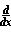Home    |    Teacher    |    Parents    |    Glossary    |    About UsEmail this page to a friendResources· Cool Tools · Formulas & Tables · References · Test Preparation · Study Tips · Wonders of MathSearchDerivative ln(x) (Math | Calculus | Derivatives | Table Of | ln)ln(x) = 1/x

Proof ofln(x) : by definition of e

Given: Definition of Derivative; Definition of e.
Solve:ln(x) = lim(d->0) [ ln(x+d) - ln(x) ] / d = lim ln((x+d)/x) / d
= lim (1/d) ln(1 + d/x) = lim [ ln (1 + d/x)^(1/d) ].

Set u=d/x and substitute:

lim(u->0) [ ln (1 + u)^(1/(ux)) ] = 1/x ln [ lim(u->0) (1 + u)^(1/u) ]
= 1/x ln (e) (Definition of e)
= 1/x.